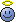# How to determine the wavefunction of photons?

• B

## Main Question or Discussion Point

Hi,

I'm sorry if this question has already been answered somewhere and I'm just too incompetent to find it, buuut:
As the title already says, I really do not get that part of quantum physics (if you can even say I'm getting ANY part at all...).
As I searched all Google for an answer I just found out about Schrödinger's equation which uses the De-Broglie wavelength, which is the analogy of the wavelength of light for matter particles. So this means Schrödinger's equation can't be used for photons, can it?
I understand that, first, every quantum particle has a wavefunction and second, the probability of finding a photon at place x is proportional to the lightwave's intensity. But, which would at least seem logical, calling the lightwave the photon's wave function doesn't seem to be correct, according to Google :D
So what am I not getting? Do photons even have a wavefunction determining their probability of presence?
The only kind of promising-looking stuff I found was Maxwell's equations, something about Dirac and some Klein-Gordon equation.
I also tried asking my teacher but we kind of always end up confusing each other...

Any help is very appreciated, thank youOh and please excuse any language mistakes, I'm not a native speakerRelated Quantum Physics News on Phys.org
hilbert2
Gold Member
I don't think the nonrelativistic Schrödinger equation is of any use in describing massless particles.

•Mathfan7
I don't think the nonrelativistic Schrödinger equation is of any use in describing massless particles.
Very well, that's indeed what I read somewhere, too.

So, is there a wavefunction for photons? I mean, there must be one, is it really enough to describe photons with the Maxwell equations? Does that count as QED?

hilbert2
Gold Member
The time-dependent Schrödinger equation for a free particle wouldn't make any sense for a massless particle, because there's a division by $m$ in the equation.

To describe photons quantum mechanically, you need QED which allows multi-photon states with an uncertain photon number. In the ordinary nonrelativistic theory, you can always tell the number of particles in any system from the number of variables the wave function depends on.

•Mathfan7
vanhees71
Gold Member
2019 Award
Well, there's not "a wave function" in relativistic QT in the same sense as there is one in non-relativistic QT. Particularly for photons the idea of a wave function a la Schrödinger is utmost misleading. The reason is very simple: At relativistic energies you can create and destroy particles (at least as long as you don't violate any conservation laws), and this cannot be adequately described by wave functions a la Schrödinger, which by construction describes one or another fixed number of particles.

For photons you need quantum-field theory (QFT), i.e., the formalism of quantized field operators, where a description of such creation and annihilation processes is very natural. Photons are particularly easily created and destroyed, because they are massless (at least to an amazing precision of emprical knowledge) and thus it's not very costly in the sense of energy to create one. It's already enough to accerate a charged particle to make "bremsstrahlung".

This QFT-formalism, however allows you (at least in principle and in an approximate sense of perturbation theory) to calculate anything we have observed today concerning electromagnetic radiation, and that's what photons really describe on the quantum level, and that's pretty similar to what's achieved in Schrödinger theory with the wave function for massive non-relativistic particles.

•Mathfan7